# NCERT Solutions For Class 8 Maths Chapter 9 Exercise 9.3 – Algebraic Expressions & Identities

NCERT Solutions for Class 8 Maths Chapter 9 Exercise 9.3 – Algebraic Expressions & Identities, has been designed by the NCERT to test the knowledge of the student on the topic – Multiplying a Monomial by a Polynomial

• Multiplying a monomial by a binomial
• Multiplying a monomial by a trinomial

### NCERT Solutions For Class 8 Maths Chapter 9 Exercise 9.3 – Algebraic Expressions & Identities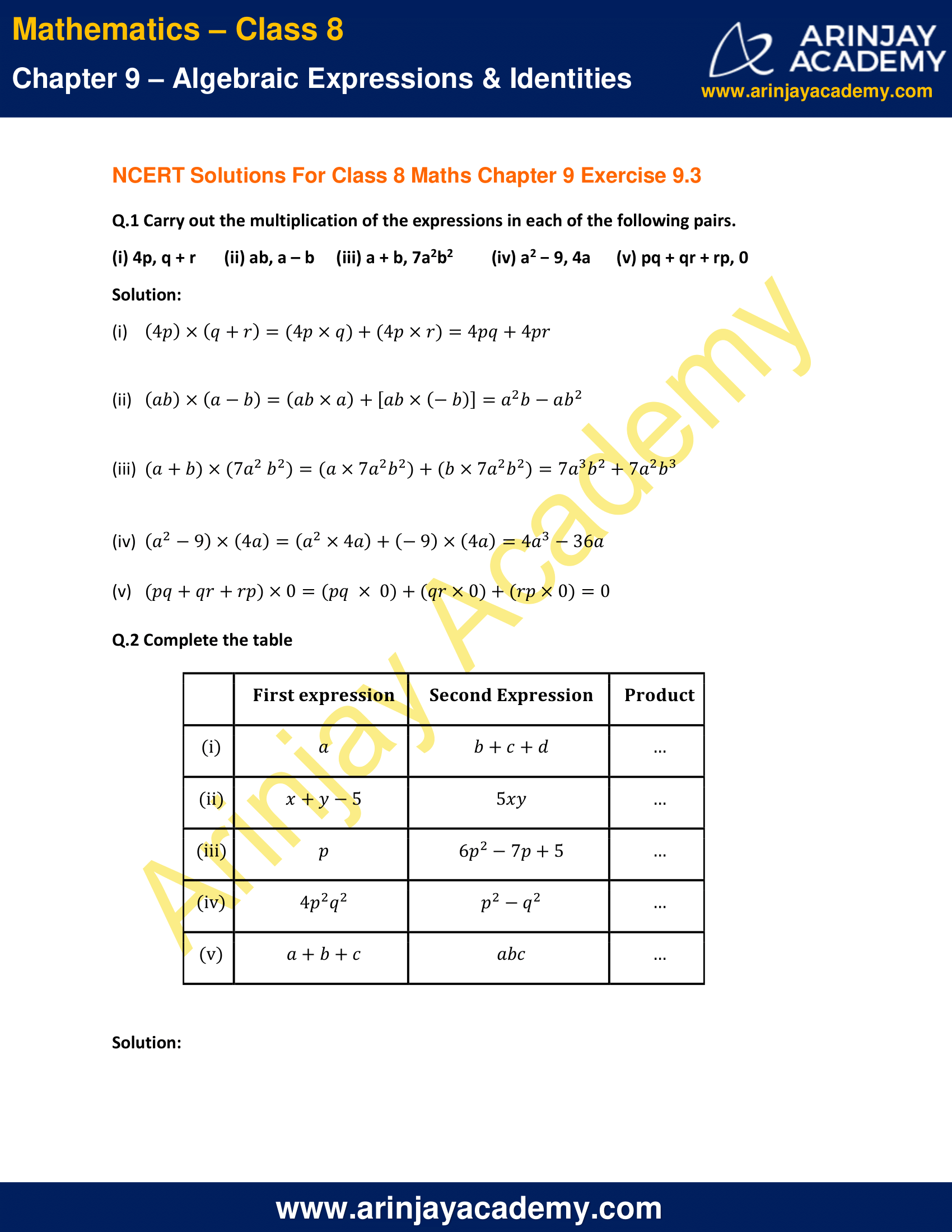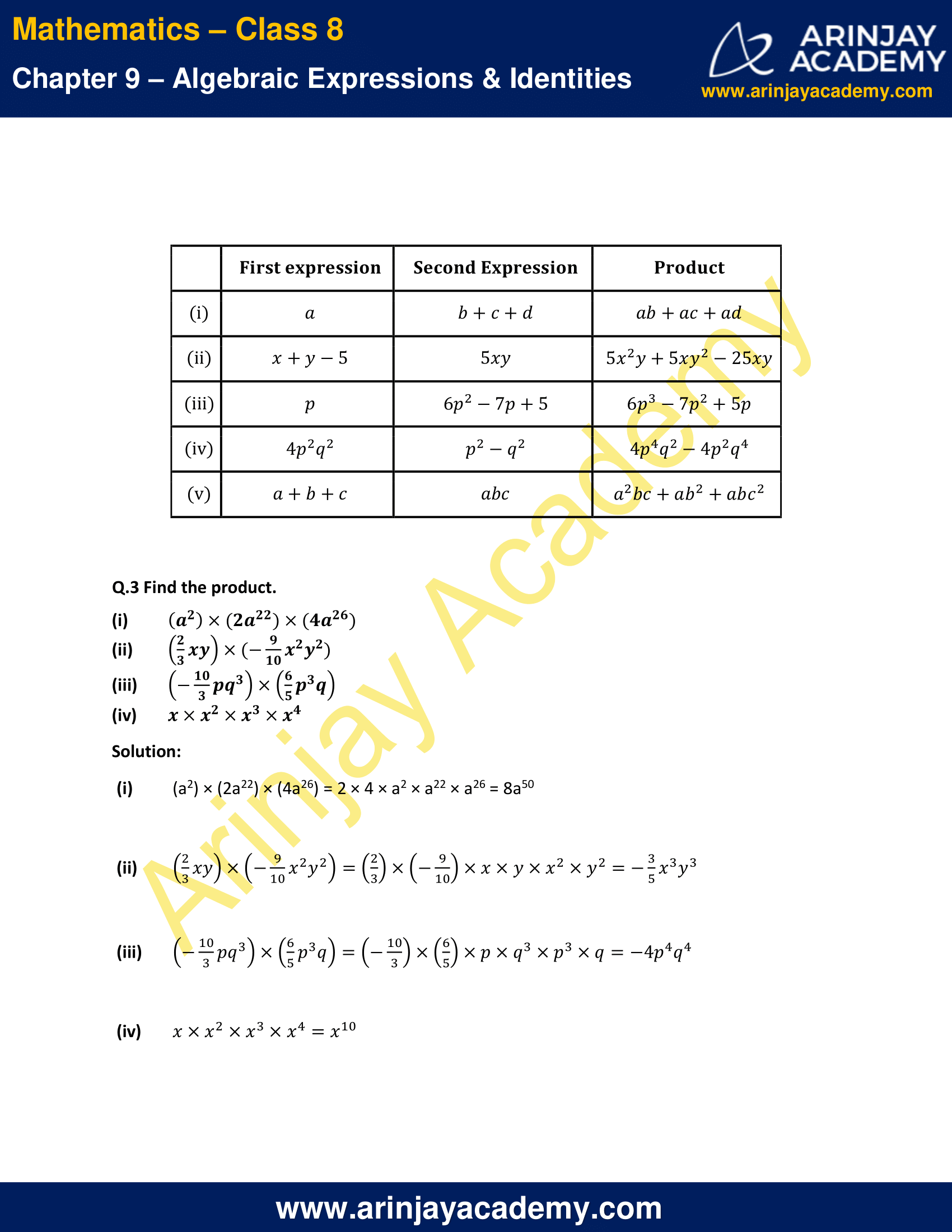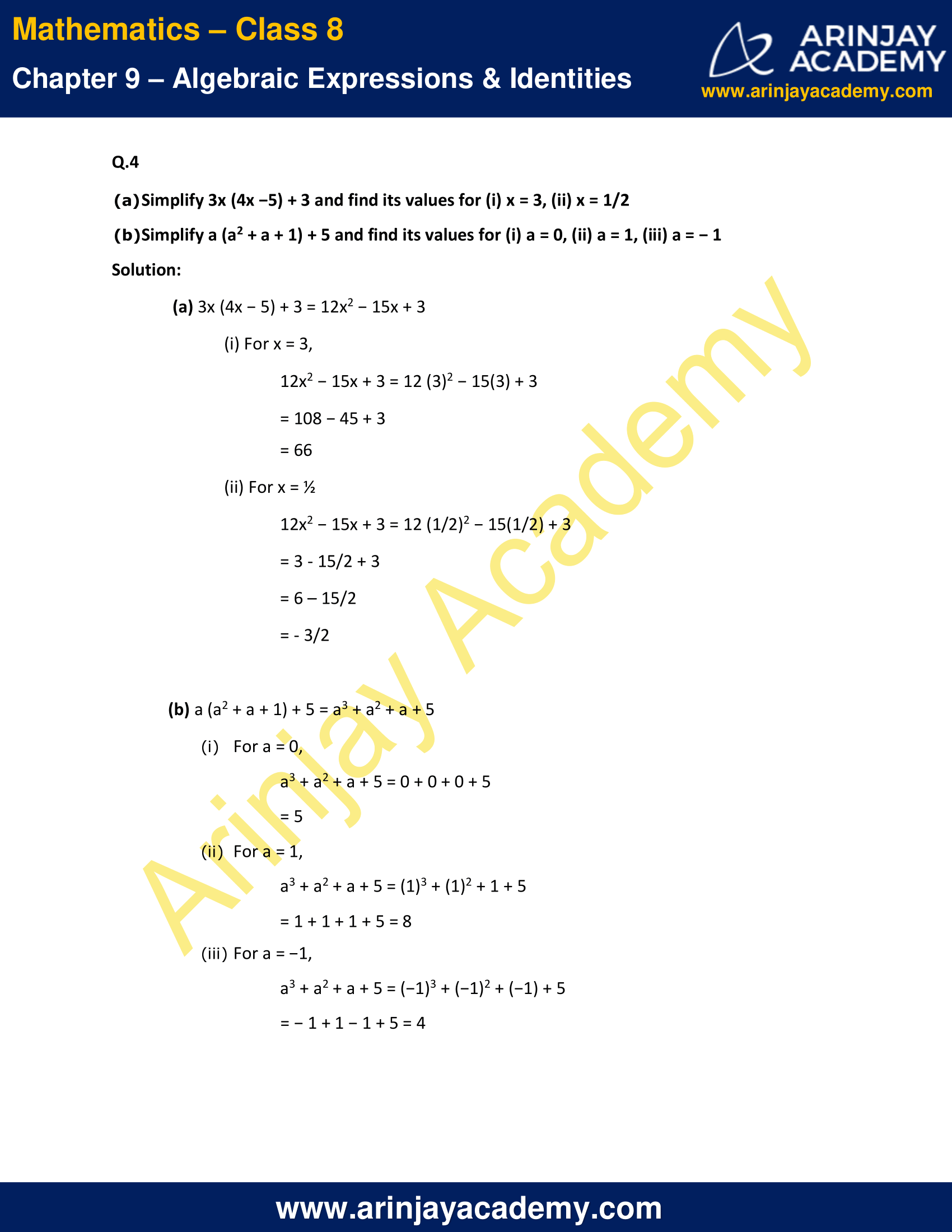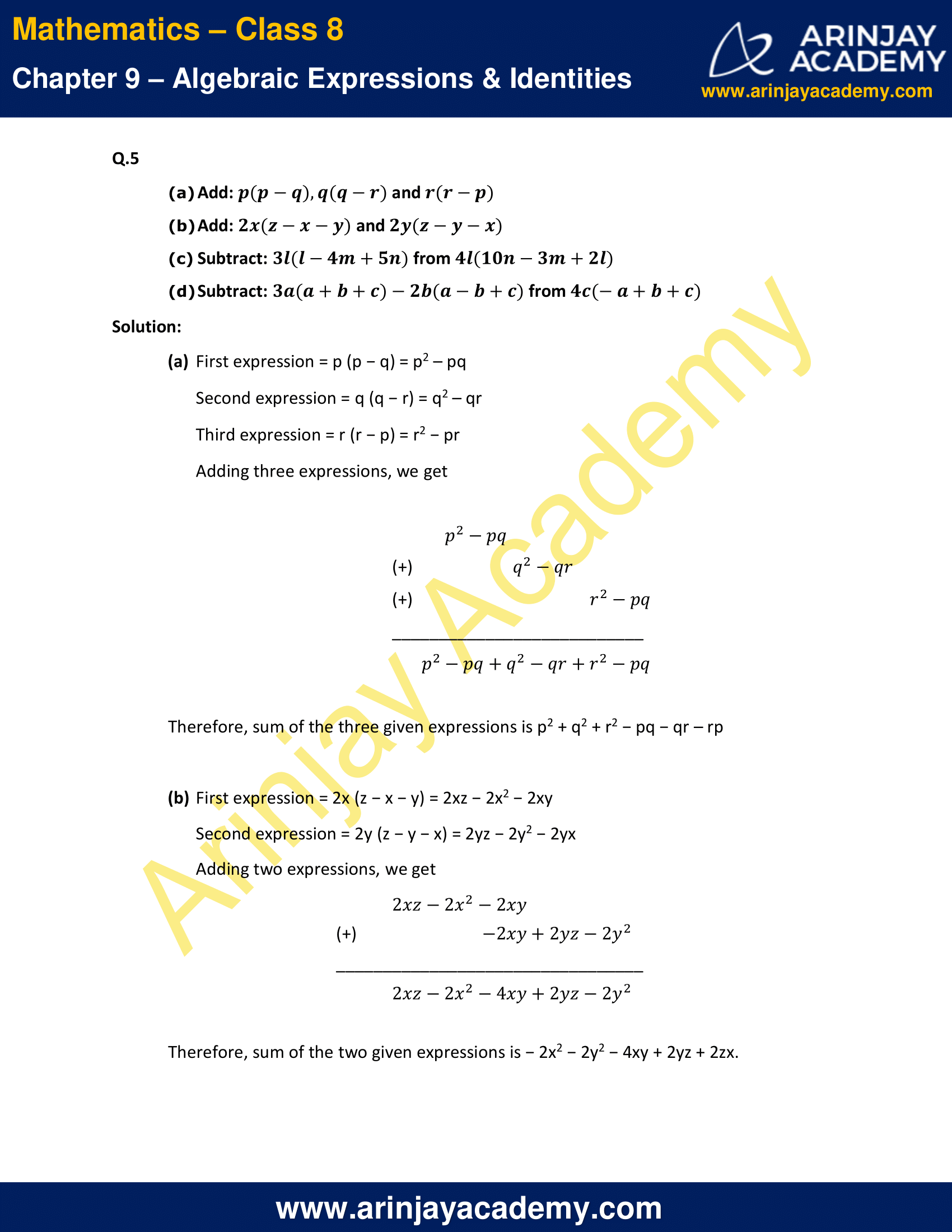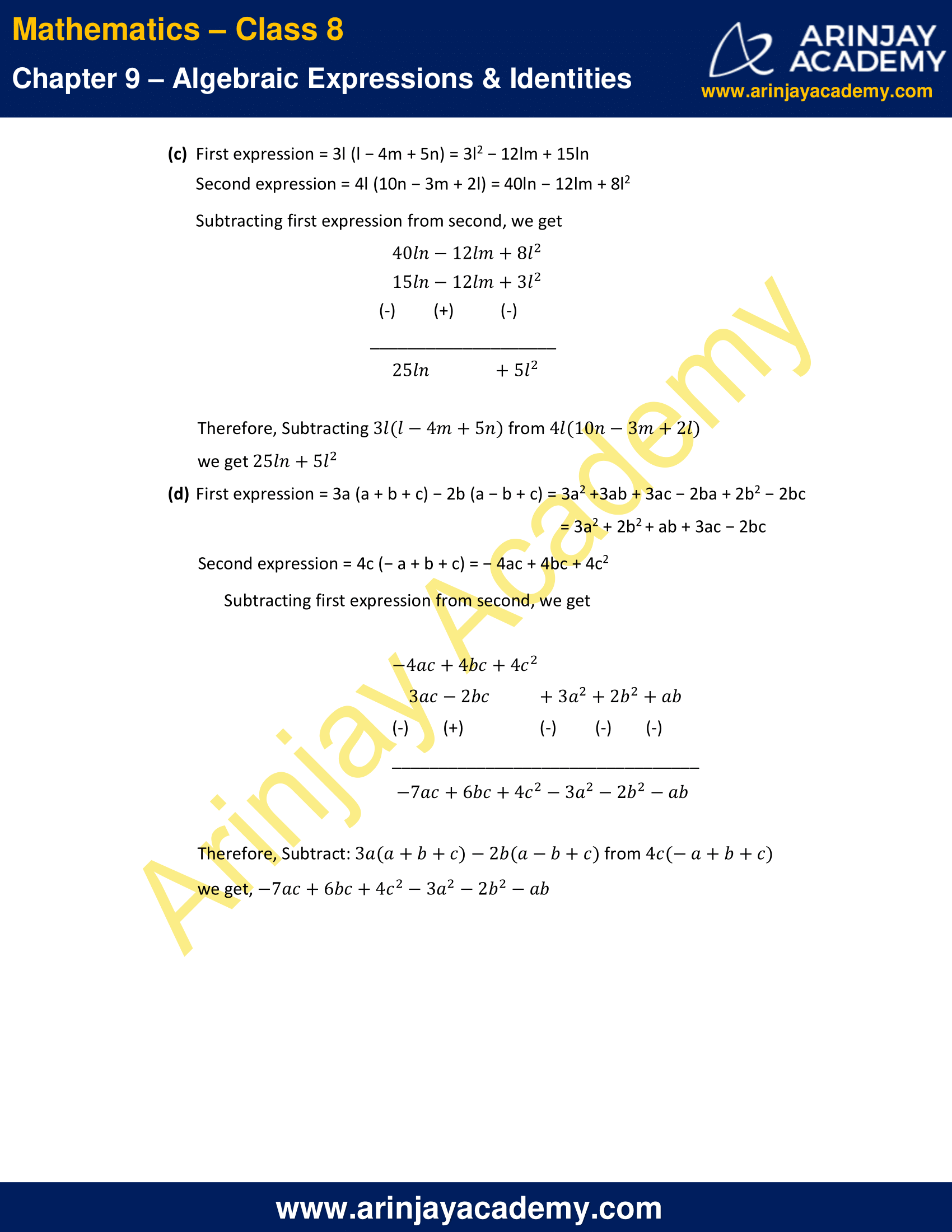NCERT Solutions For Class 8 Maths Chapter 9 Exercise 9.3 – Algebraic Expressions & Identities

Q.1 Carry out the multiplication of the expressions in each of the following pairs.

(i) 4p, q + r
(ii)
ab, ab
(iii)
a + b, 7a2b2
(iv)
a2 − 9, 4a
(v)
pq + qr + rp, 0

Solution:

(i) (4p)×(q+r)=(4p×q)+(4p×r)=4pq+4pr
(ii) (ab)×(a-b)=(ab×a)+[ab×(- b)]=a²b-ab²
(iii) (a+b)×(7a²b²)=(a×7a²b²)+(b×7a²b²)=7a³b²+7a²b³
(iv) (a²-9)×(4a)=(a²×4a)+(- 9)×(4a)=4a³-36a
(v) (pq+qr+rp)×0=(pq × 0)+(qr×0)+(rp×0)=0

Q.2 Complete the table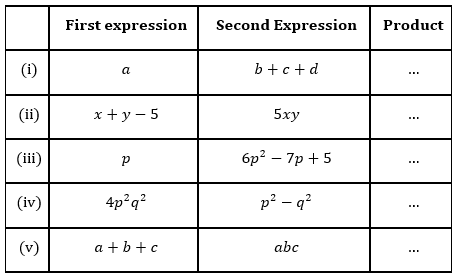Solution: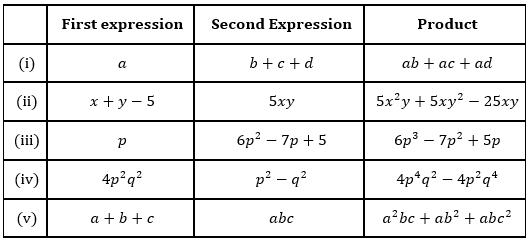Q.3 Find the product.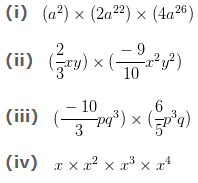Solution: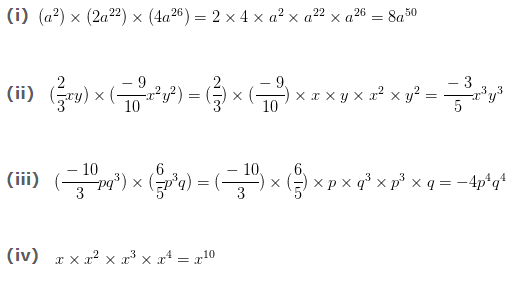Q.4

(a) Simplify 3x (4x −5) + 3 and find its values for (i) x = 3, (ii) x = 1/2

(b) Simplify a (a2 + a + 1) + 5 and find its values for (i) a = 0, (ii) a = 1, (iii) a = − 1

Solution:

(a) 3x (4x − 5) + 3 = 12x2 − 15x + 3

(i) For x = 3,

12x2 − 15x + 3 = 12 (3)2 − 15(3) + 3

= 108 − 45 + 3

= 66

(ii) For x = ½

12x2 − 15x + 3 = 12 (1/2)2 − 15(1/2) + 3

= 3 – 15/2 + 3

= 6 – 15/2

= – 3/2

(b) a (a2 + a + 1) + 5 = a3 + a2 + a + 5

(i) For a = 0,

a3 + a2 + a + 5 = 0 + 0 + 0 + 5

= 5

(ii) For a = 1,

a3 + a2 + a + 5 = (1)3 + (1)2 + 1 + 5

= 1 + 1 + 1 + 5 = 8

(iii) For a = −1,

a3 + a2 + a + 5 = (−1)3 + (−1)2 + (−1) + 5

= − 1 + 1 − 1 + 5 = 4

Q.5
(c) Subtract: 3l(l-4m+5n) from 4l(10n-3m+2l)
(d) Subtract: 3a(a+b+c)-2b(a-b+c) from 4c(- a+b+c)

Solution:

(a) First expression = p (p − q) = p2 – pq
Second expression = q (q − r) = q2 – qr
Third expression = r (r − p) = r2 − pr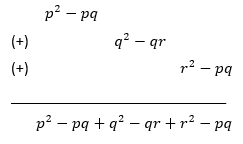Therefore, sum of the three given expressions is p2 + q2 + r2 − pq − qr – rp

(b) First expression = 2x (z − x − y) = 2xz − 2x2 − 2xy
Second expression = 2y (z − y − x) = 2yz − 2y2 − 2yx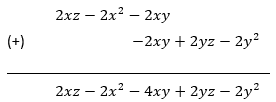Therefore, sum of the two given expressions is − 2x2 − 2y2 − 4xy + 2yz + 2zx.

(c) First expression = 3l (l − 4m + 5n) = 3l2 − 12lm + 15ln
Second expression = 4l (10n − 3m + 2l) = 40ln − 12lm + 8l2
Subtracting first expression from second, we get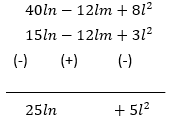Therefore, Subtracting 3l(l-4m+5n) from 4l(10n-3m+2l)
we get 25ln+5l²

(d) First expression = 3a (a + b + c) − 2b (a − b + c) = 3a2 +3ab + 3ac − 2ba + 2b2 − 2bc
= 3a2 + 2b2 + ab + 3ac − 2bc
Second expression = 4c (− a + b + c) = − 4ac + 4bc + 4c2
Subtracting first expression from second, we get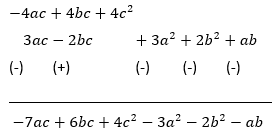Therefore, Subtract: 3a(a+b+c)-2b(a-b+c) from 4c(- a+b+c)
we get, -7ac+6bc+4c²-3a²-2b²-ab

The next Exercise for NCERT Solutions for Class 8 Maths Chapter 9 Exercise 9.4 – Algebraic Expressions and Identities can be accessed by clicking here

NCERT Solutions For Class 8 Maths Chapter 9 Exercise 9.3 – Algebraic Expressions and Identities